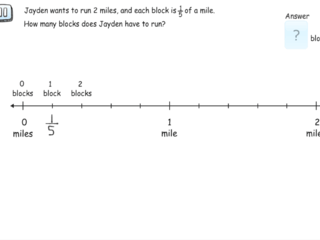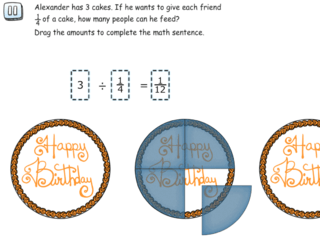# Interactive Problem Bank

•Fraction Circles
•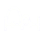Number Lines
•Fraction Bars
•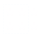Sets
•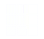Area Models
•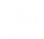Base Ten Blocks
By TopicBy Standard
• 5.NBT.2
• 5.NF.1
• 5.NF.2
• 5.NF.3
• 5.NF.4a
• 5.NF.4b
• 5.NF.5
• 5.NF.6
• 5.NF.7a
• 5.NF.7b
• 5.NF.7c
Can't find what you're looking for? Send us a note!

## Grade 5 - 5.NBT - Number and Operations in Base Ten

### 5.NBT.2

Explain patterns in the number of zeros of the product when multiplying a number by powers of 10, and explain patterns in the placement of the decimal point when a decimal is multiplied or divided by a power of 10. Use whole-number exponents to denote powers of 10.

#### Decimal Point Patterns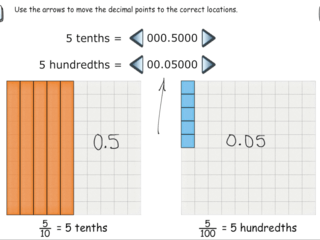## Grade 5 - 5.NF - Number and Operations - Fractions

### 5.NF.1

Add and subtract fractions with unlike denominators (including mixed numbers) by replacing given fractions with equivalent fractions in such a way as to produce an equivalent sum or difference of fractions with like denominators. For example, 2/3 + 5/4 = 8/12 + 15/12 = 23/12. (In general, a/b + c/d = (ad + bc)/bd.)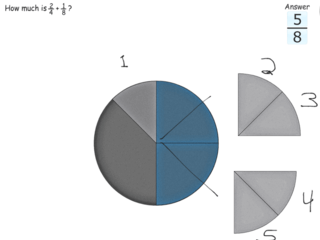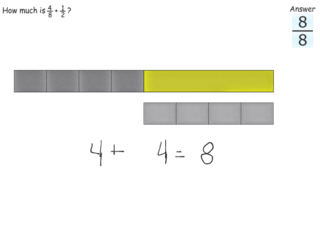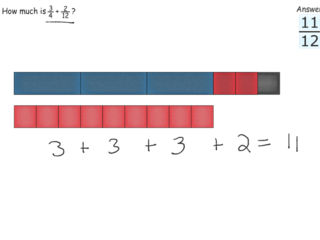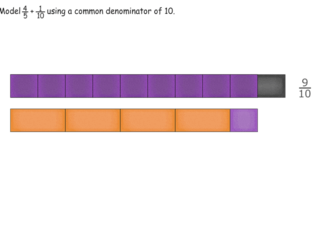#### Adding Proper Fractions Using Models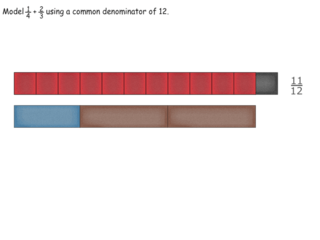#### Subtracting Fractions Using Models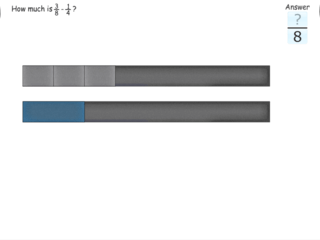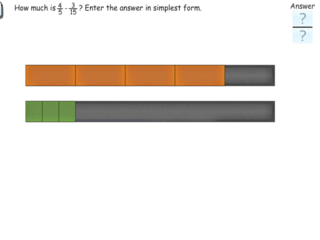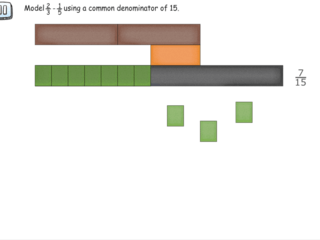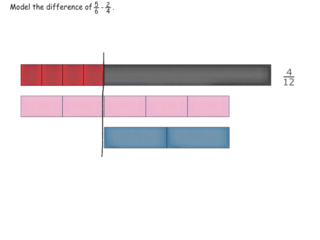#### Subtracting Fractions Using Number Line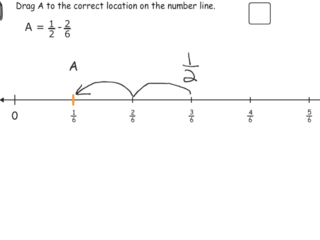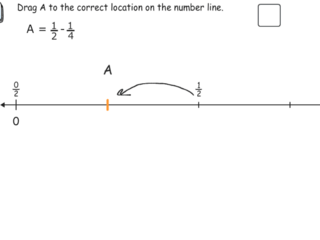#### Borrowing with Common Denominators on the Number Line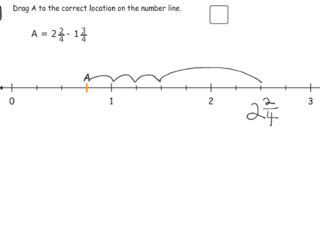### 5.NF.2

Solve word problems involving addition and subtraction of fractions referring to the same whole, including cases of unlike denominators, e.g., by using visual fraction models or equations to represent the problem. Use benchmark fractions and number sense of fractions to estimate mentally and assess the reasonableness of answers. For example, recognize an incorrect result 2/5 + 1/2 = 3/7, by observing that 3/7 < 1/2.

#### Estimate Sums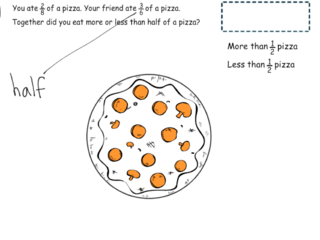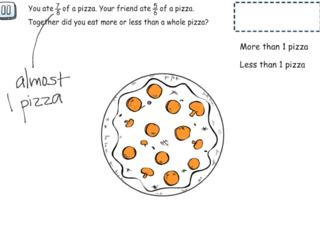#### Subtracting Fractions from Whole Numbers on Number Line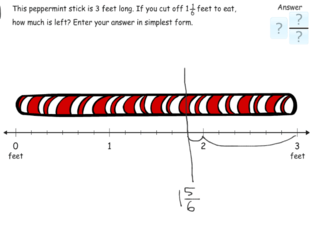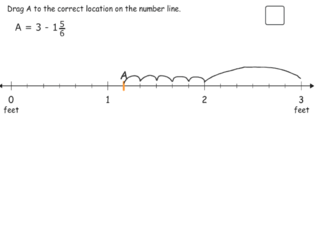### 5.NF.3

Interpret a fraction as division of the numerator by the denominator (a/b = a ÷ b). Solve word problems involving division of whole numbers leading to answers in the form of fractions or mixed numbers, e.g., by using visual fraction models or equations to represent the problem. For example, interpret 3/4 as the result of dividing 3 by 4, noting that 3/4 multiplied by 4 equals 3, and that when 3 wholes are shared equally among 4 people each person has a share of size 3/4. If 9 people want to share a 50-pound sack of rice equally by weight, how many pounds of rice should each person get? Between what two whole numbers does your answer lie?

#### Interpreting and Expressing a/b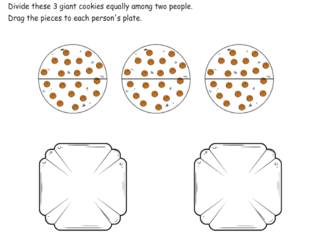#### Interpreting and Expressing a/b when a>b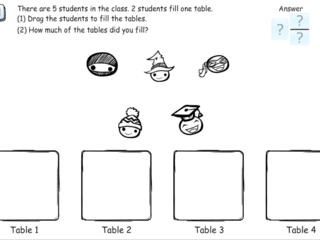#### Estimating Quotients of Whole Numbers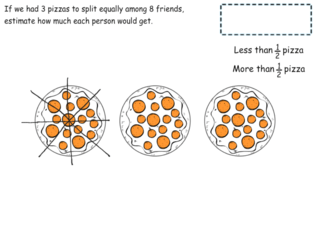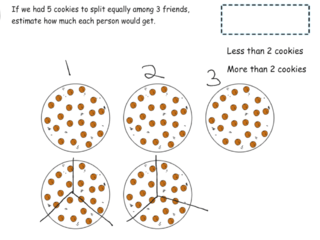#### Whole Number Divisors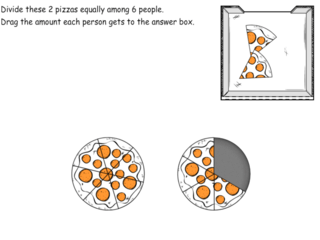### 5.NF.4a

Interpret the product (a/b) × q as a parts of a partition of q into b equal parts; equivalently, as the result of a sequence of operations a × q ÷ b. For example, use a visual fraction model to show (2/3) × 4 = 8/3, and create a story context for this equation. Do the same with (2/3) × (4/5) = 8/15. (In general, (a/b) × (c/d) = ac/bd.)

#### Multiplication of Whole Numbers by Mixed Numbers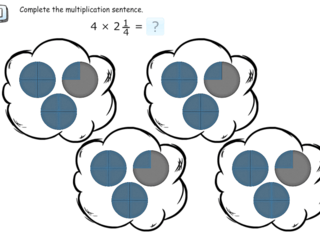#### Multiplication of Mixed Numbers by Whole Numbers Using Number Lines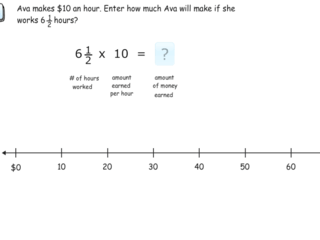#### Multiplication of Fractions Using Models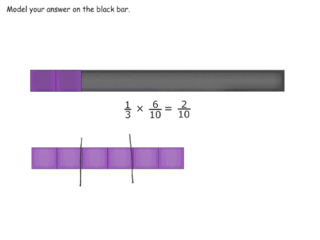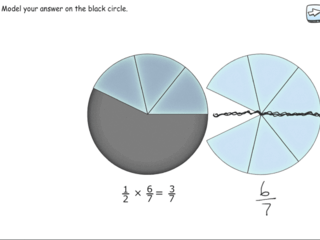### 5.NF.4b

Find the area of a rectangle with fractional side lengths by tiling it with unit squares of the appropriate unit fraction side lengths, and show that the area is the same as would be found by multiplying the side lengths. Multiply fractional side lengths to find areas of rectangles, and represent fraction products as rectangular areas.

#### Multiplication of a Fraction by a Fraction Using Area Model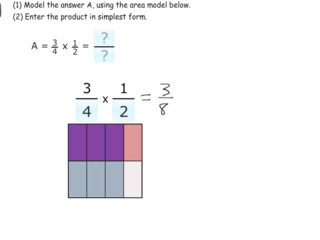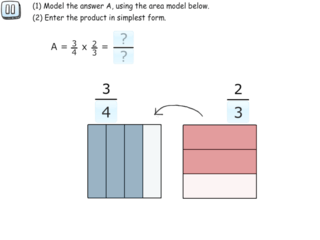### 5.NF.5

Interpret multiplication as scaling (resizing), by:
1. Comparing the size of a product to the size of one factor on the basis of the size of the other factor, without performing the indicated multiplication.
2. Explaining why multiplying a given number by a fraction greater than 1 results in a product greater than the given number (recognizing multiplication by whole numbers greater than 1 as a familiar case); explaining why multiplying a given number by a fraction less than 1 results in a product smaller than the given number; and relating the principle of fraction equivalence a/b = (n × a)/(n × b) to the effect of multiplying a/b by 1.

### 5.NF.6

Solve real world problems involving multiplication of fractions and mixed numbers, e.g., by using visual fraction models or equations to represent the problem.

#### Multiplication of Fractions and Mixed Numbers### 5.NF.7a

Interpret division of a unit fraction by a non-zero whole number, and compute such quotients. For example, create a story context for (1/3) ÷ 4, and use a visual fraction model to show the quotient. Use the relationship between multiplication and division to explain that (1/3) ÷ 4 = 1/12 because (1/12) × 4 = 1/3.

#### Modeling Unit Fractions Divided by Whole Numbers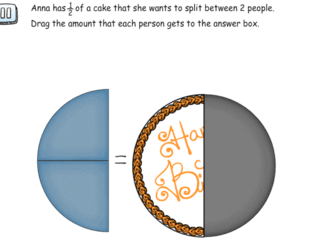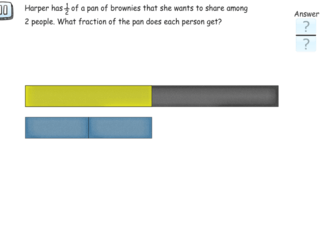#### Unit Fractions Divided by Whole Numbers on a Number Line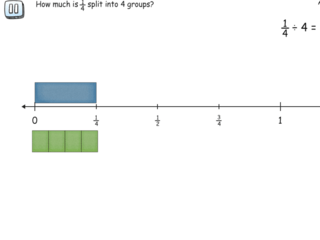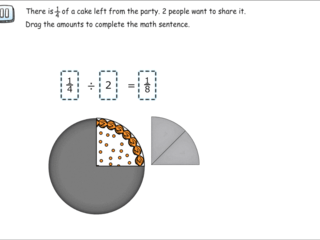#### Unit Fractions Divided by Whole Numbers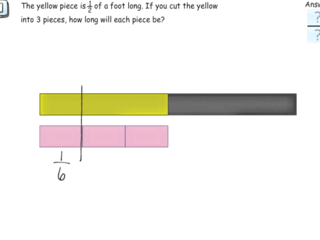### 5.NF.7b

Interpret division of a whole number by a unit fraction, and compute such quotients. For example, create a story context for 4 ÷ (1/5), and use a visual fraction model to show the quotient. Use the relationship between multiplication and division to explain that 4 ÷ (1/5) = 20 because 20 × (1/5) = 4.

#### Modeling Whole Numbers Divided by Unit Fractions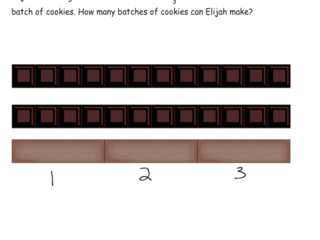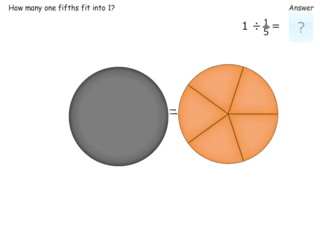#### Understanding Division as the Inverse of Multiplication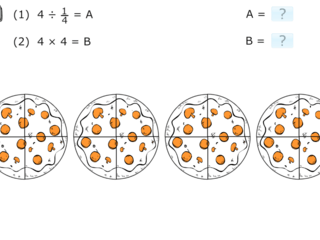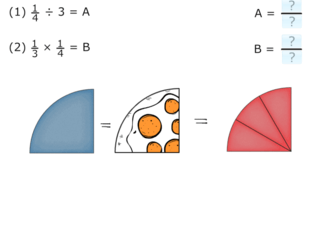### 5.NF.7c

Solve real world problems involving division of unit fractions by non-zero whole numbers and division of whole numbers by unit fractions, e.g., by using visual fraction models and equations to represent the problem. For example, how much chocolate will each person get if 3 people share 1/2 lb of chocolate equally? How many 1/3-cup servings are in 2 cups of raisins?

#### Modeling Whole Numbers Divided by Unit Fractions